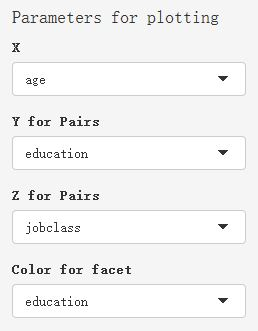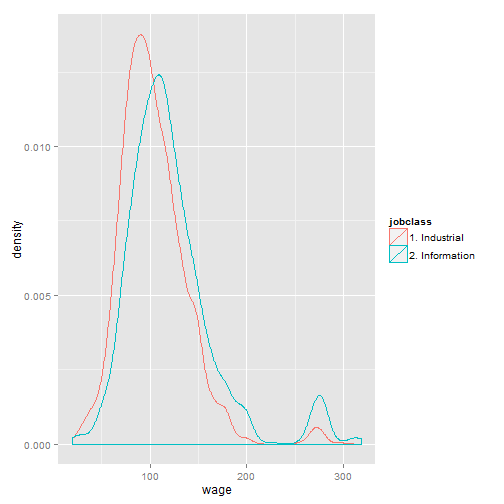# Exploration of Wage

Xiaojing HU
Sat Jun 20 21:47:16 2015

### About the Shiny Application

The application uses Wage dataset in library ISLR for you to explore the relationship between wage and other variables.

There is a plot about the distribution of wage, with the mean in red line, on the top of webage.
The default value of the wage slidebar on the left is the median of wage. You can move the slidebar to see the value in plot (blue line).

### Plot Type

The main part is three types of plotting:

1. Pairs: Comparing wage with other three variables at once.
2. Points: Relationship between wage and one selected variable grouped by another selected variable.
3. Density: Wage density grouped by one selected variable.

### Parameters for plottingSelect Parameters for plotting to explore the relationship. Parameters are variables in Wage dataset:

• X is used in all three types of plots
• Y and Z are used in Pairs plot
• Color for facet is used in Points and Density plots.

### Plot Example

• Density Plot
• grouped by jobclass
library(ggplot2)
library(ISLR)
data(Wage)
qplot(wage, color=jobclass, data=Wage, geom="density")